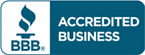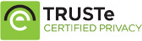# Solution manual for Structural Dynamics Theory and Applications by Joseph W. Tedesco

\$30.00

Structural Dynamics Theory and Applications by Joseph W. Tedesco Answers for Questions

Full Chapters are included## Description

Solution manual for Structural Dynamics Theory and Applications by Joseph W. Tedesco

Basic Concepts
Introduction to Structural Dynamics
Types of Dynamic Loads
Sources of Dynamic Loads
Distinguishing Features of a Dynamic Problem
Methodology for Dynamic Analysis
Types of Structural Vibration
Organization of the Text
Systems of Units
References
Single-Degree-of-Freedom (SDOF) Systems
Equation of Motion and Natural Frequency
Fundamental Components of a Vibrating System
D’Alembert’s Principle of Dynamic Equilibrium
The Energy Method
The Principle of Virtual Displacements
References
Notation
Problems
Undamped Free Vibration
Simple Harmonic Motion
Interpretation of the Solution
Equivalent Stiffness
Rayleigh Method
References
Notation
Problems
4
Free Vibration with Viscous Damping
Logarithmic Decrement
Hysteresis Damping
Coulomb Damping
References
Notation
Problems
Response to Harmonic Excitation
Forced Harmonic Response of Undamped Systems
Beating and Resonance
Forced Harmonic Vibrations with Viscous Damping
Effect of Damping Factor on Steady-State Response and Phase Angle
Harmonic Excitation Caused by Rotating Unbalance
Base Excitation
Vibration Isolation and Transmissibility
References
Notation
Problems
Response to Periodic and Arbitrary Dynamic Excitation
Response to Periodic Excitation
Response to Unit Impulse
Duhamel Integral
Response to Arbitrary Dynamic Excitation
Response Spectrum
References
Notation
Problems
Numerical Evaluation of Dynamic Response
Interpolation of the Excitation
Direct Integration of the Equation of Motion
Central Difference Method
Runge-Kutta Methods
Average Acceleration Method
Linear Acceleration Method
Response to Base Excitation
Response Spectra by Numerical Integration
References
Notation
Problems
Frequency Domain Analysis
Alternative Forms of the Fourier Series
Discrete Fourier Transform
Fast Fourier Transform
Discrete Fourier Transform Implementation Considerations
Fourier Integral
References
Notation
Problems
Multi-Degree-of-Freedom (MDOF) Systems
General Property Matrices for Vibrating Systems
Flexibility Matrix
Stiffness Matrix
Inertia Properties: Mass Matrix
The Eigenproblem in Vibration Analysis
Static Condensation of the Stiffness Matrix
References
Notation
Problems
Equations of Motion and Undamped Free Vibration
Hamilton’s Principle and the Lagrange Equations
Natural Vibration Frequencies
Natural Vibration Modes
Orthogonality of Natural Modes
Systems Admitting Rigid-Body Modes
Generalized Mass and Stiffness Matrices
Free Vibration Response to Initial Conditions
Approximate Methods for Estimating the Fundamental Frequency
References
Notation
Problems
Numerical Solution Methods for Natural Frequencies and Mode Shapes
General Solution Methods for Eigenproblems
Inverse Vector Iteration
Forward Vector Iteration
Generalized Jacobi Method
Solution Methods for Large Eigenproblems
References
Notation
Problems

## Reviews

There are no reviews yet.